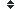fraction (28 synonym found)

### Sort fraction by:    Alphabetically| Length| Random | DefinitionDefinition of fraction:

(n) : a part of a whole, especially a comparatively small part; a ratio of two integers, the numerator and the denominator, usually written one above the other and separated by a vinculum (horizontal bar)
(v) : divide or break into fractions
History
This word has been
viewed 1,758 times.

Related searches
for fraction:

Most Recent

Definition of fraction:

(n) : a part of a whole, especially a comparatively small part; a ratio of two integers, the numerator and the denominator, usually written one above the other and separated by a vinculum (horizontal bar)
(v) : divide or break into fractions